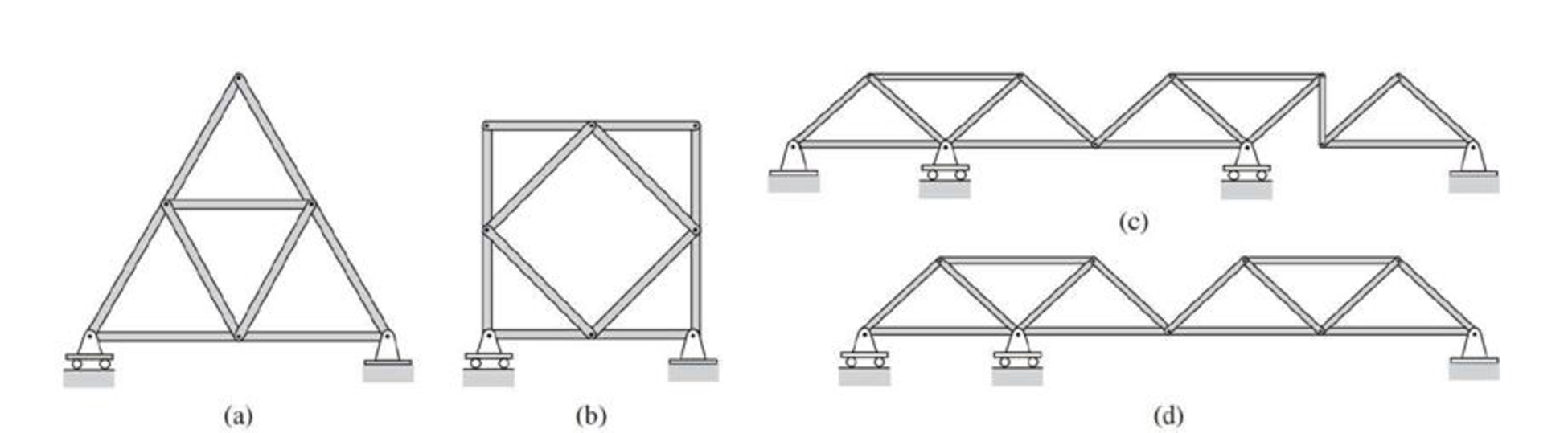# Classify each of the plane trusses shown as unstable, statically determine or statically indeterminate. FIG.P4.5

#### Solutions

Chapter
Section
Chapter 4, Problem 5P
Textbook Problem
31 views

## Classify each of the plane trusses shown as unstable, statically determine or statically indeterminate.FIG.P4.5

(a)

To determine

Categorize the given plane truss as unstable, statically indeterminate or statically determinate.

Find the degree of static indeterminacy in case the given truss is statically indeterminate.

### Explanation of Solution

Given information:

The Figure of plane truss is shown.

Calculation:

Show the conditions for plane truss as follows:

m+r<2jthe truss is statically unstablem+r=2jthe truss is statically determinatem+r>2jthe truss is statically indeterminate}        (1)

Here, m is the number of members of the truss, r is the support reactions and j is the number of joints

(b)

To determine

Categorize the given plane truss as unstable, statically indeterminate or statically determinate.

Find the degree of static indeterminacy in case the given truss is statically indeterminate.

(c)

To determine

Categorize the given plane truss as unstable, statically indeterminate or statically determinate.

Find the degree of static indeterminacy in case the given truss is statically indeterminate.

(d)

To determine

Categorize the given plane truss as unstable, statically indeterminate or statically determinate.

Find the degree of static indeterminacy in case the given truss is statically indeterminate.

### Still sussing out bartleby?

Check out a sample textbook solution.

See a sample solution

#### The Solution to Your Study Problems

Bartleby provides explanations to thousands of textbook problems written by our experts, many with advanced degrees!

Get Started

Find more solutions based on key concepts
For Problems 16.1 through 16.19, draw the top, the front, and the right-side orthographic views of the objects ...

Engineering Fundamentals: An Introduction to Engineering (MindTap Course List)

Check the adequacy of the bolts. The given loads are service loads. a. Use LRFD. b. Use ASD.

Steel Design (Activate Learning with these NEW titles from Engineering!)

What are some elements that are typically included in network diagrams?

Network+ Guide to Networks (MindTap Course List)

drive a. operating system problem that occurs when it spends much of its time paging, instead of executing appl...

Enhanced Discovering Computers 2017 (Shelly Cashman Series) (MindTap Course List)

If your motherboard supports ECC DDR3 memory, can you substitute non-ECC DDR3 memory?

A+ Guide to Hardware (Standalone Book) (MindTap Course List)

What is the maximum width of the cover pass? Why?

Welding: Principles and Applications (MindTap Course List)